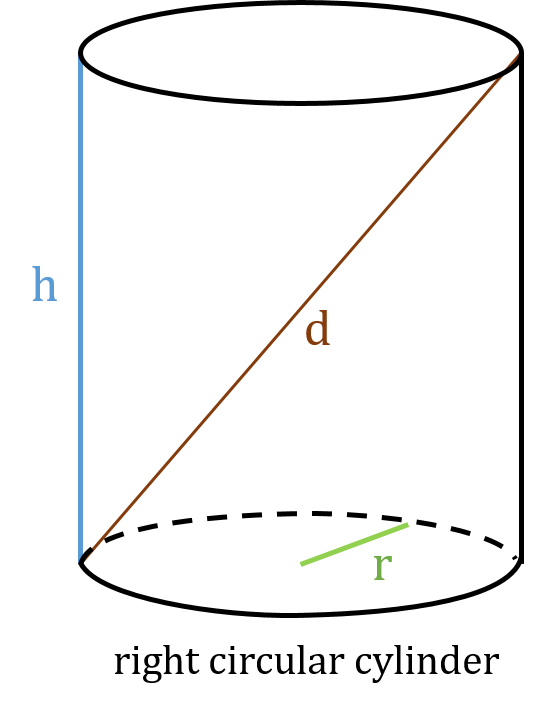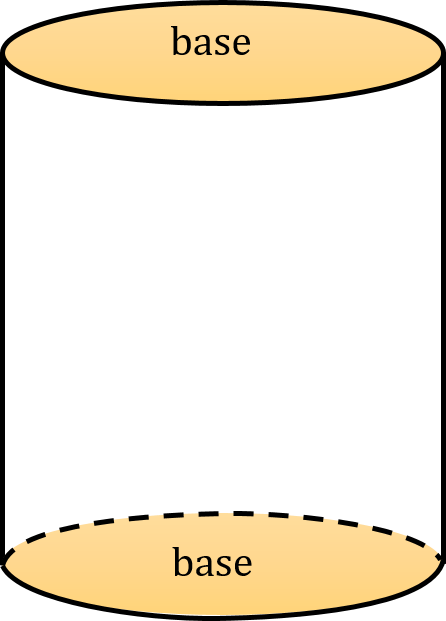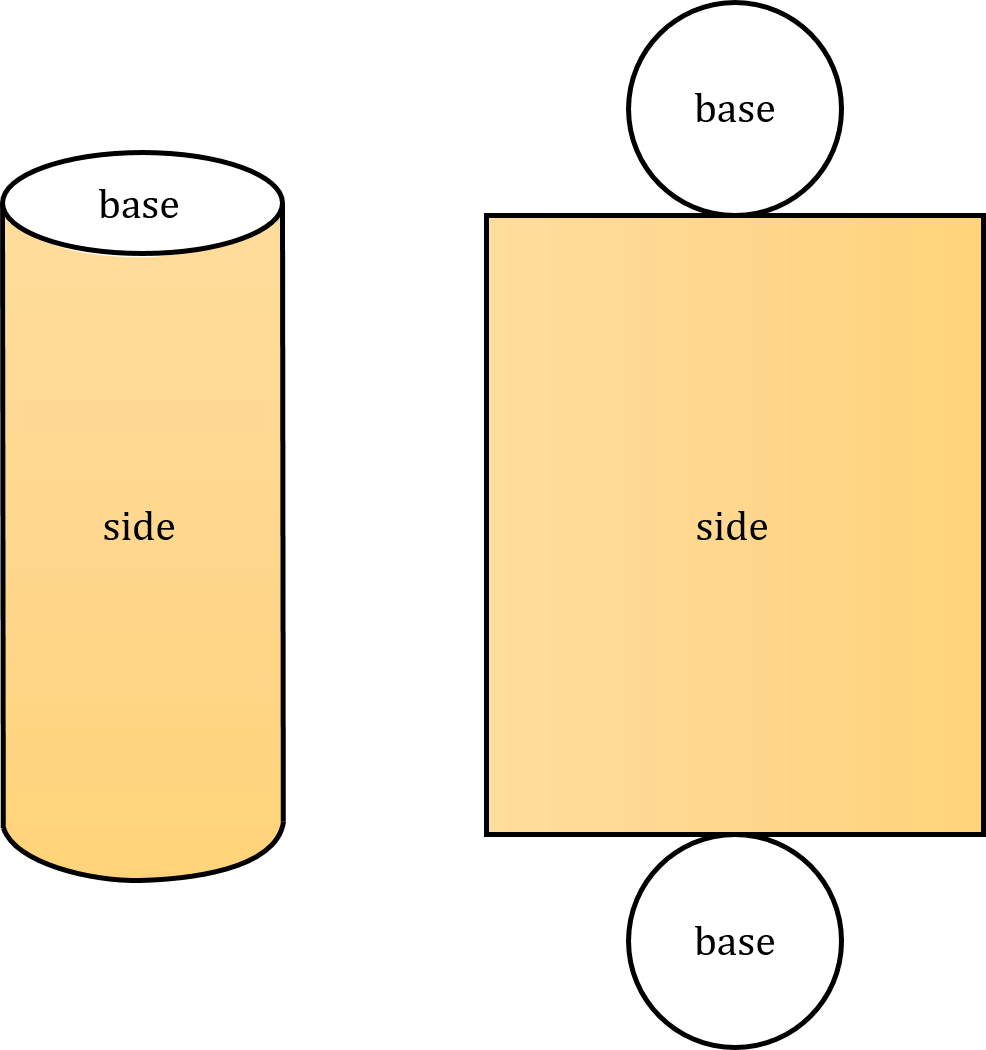# Right Cylinder Calc: find A, V, A_l, A_b

Created by Dominik Czernia, PhD
Reviewed by Bogna Szyk and Adena Benn
Last updated: Feb 11, 2023

Right cylinder calc is an advanced tool that finds the area, volume, lateral area and base area of a right cylinder. Enter some of your selected values to calculate other parameters or keep reading the article below to learn about all the equations we have used. The notation is as follows:• r – Radius of a circle (base);
• h – Height of a cylinder;
• A – Total surface area of a cylinder (right cylinder calc: find A);
• V – Volume of a cylinder (right cylinder calc: find V);
• A_l – Lateral surface area of a cylinder (right cylinder calc: find A_l);
• A_b – Base surface area of a cylinder (right cylinder calc: find A_b);
• d – Longest diagonal of a cylinder; and
• A / V – Surface-to-volume ratio of a cylinder.

A cylinder is a three-dimensional solid with two congruent parallel planes called bases of the cylinder. It consists of all the points on all the lines that connect its bases. A cylinder is right when one base lies exactly above the other. Otherwise, it is called an oblique cylinder. If the bases are circles, then the cylinder is called a circular cylinder. Remember that traditionally, the term cylinder usually refers to the right circular cylinder – our right cylinder calc is dedicated to this type of cylinder.

You should also check out our cylindrical coordinates calculator and see how you can use a cylinder to describe the position of any given point in a 3D space.

## Right cylinder calc: find A_bThe base surface area of a right circular cylinder A_b is simply the sum of the areas of two bases. Its value is expressed in squared units of length, e.g., square meters m² or square feet ft². Check out area conversion calculator to learn how to convert between different area units! Because both bases of a right circular cylinder are the same circles with the radius r, the base surface area of a cylinder is two times the area of a circle:

a_b = 2 × π × r².

But what if you don't know the radius of the base? There are several different ways to estimate the base surface area of a cylinder:

1. With given total area and lateral area:

A_b = A - A_l.

2. With given volume and height:

A_b = 2 × V / h.

3. With given lateral area and height:

A_b = A_l²/(2 × π × h²).

4. With given longest diagonal and height:

A_b = π/2 × (d² - h²).

## Right cylinder calc: find A_l

To find the lateral surface area of a right circular cylinder A_l, we open the cylinder like a carton box and flatten it out to find out which geometric figures it contains – this is the so-called net of a solid. As you can see below, a right circular cylinder consists of two circles (bases) and one rectangle (lateral surface).The first side of that rectangle is the height h of the cylinder, and the second side equals the circumference of the base – 2 × π × r (circle with radius r), as shown in the circumference calculator. Therefore, the lateral surface area of a right circular cylinder A_l can be found just like the area of a rectangle:

A_l = 2 × π × r × h.

There are also other equations that lead to the lateral surface area of a cylinder:

1. With given total area and base area:

A_l = A - A_b

2. With given volume and height:

A_l = √(4 × π × h × V)

3. With given base area and height:

A_l = √(2 × π × h² × A_b)

4. With given longest diagonal and height:

A_l = √(d²h²π² - h⁴π²)

If you need to make several calculations with rectangles, then our area of a rectangle calculator might be the right tool to check!

## Right cylinder calc: find A

The total surface area of a right circular cylinder A is the sum of all the areas of the faces that a cylinder has, i.e., a top base, a bottom base, and a side. Therefore, the total surface area of a right circular cylinder is the sum of its base surface area A_b and its lateral surface area A_l:

A = A_b + A_l = 2 × π × r² + 2 × π × r × h

and after some trivial simplification

A = 2 × π × r × (r + h)

## Right cylinder calc: find V

The volume of a right circular cylinder V is the space enclosed by a cylinder. Its value is expressed in cubic units of length, e.g., cubic meters m³ or cubic feet cu ft. Try our volume conversion to learn how to convert between different volume units! The volume of a right circular cylinder is a product of the height h and the area of one base A_b/2 = π × r². Thus, it can be found with the following equation:

V = A_b/2 × h = π × h × r²

It is worth remembering that this formula holds whether or not the cylinder is a right cylinder – it is also true for oblique cylinders. In the case of a right circular cylinder, you can also use other formulas to estimate the volume of a cylinder:

1. With given lateral area and height:

V = A_l² / (4 × π × h),

2. With given lateral area and radius:

V = A_l × r / 2,

3. With given base area and lateral area:

V = √(A_l² × A_b / (8 × π)),

4. With given longest diagonal and height:

V = (π × h × d² - π × h³)/4.

Dominik Czernia, PhDin
Height (h)
in
Surface areas
Base surface area (A_b)
in²
Lateral surface area (A_l)
in²
Total surface area (A)
in²
Volume
Volume (V)
cu in
People also viewed…

Addiction calculator tells you how much shorter your life would be if you were addicted to alcohol, cigarettes, cocaine, methamphetamine, methadone, or heroin.

### Right triangle trigonometry

Discover the relationship between trigonometry and triangles with Omni's right triangle trigonometry calculator!

### Snowman

The perfect snowman calculator uses math & science rules to help you design the snowman of your dreams!

### Surface area of a cone

How to find the surface area of a cone? What's the surface area of a cone formula? Click here to find out.